# How to check if a number is a Perfect Square

Squares of all integers are known as perfect squares. In this lesson, we will discuss a very interesting Mathematical shortcut: How to check whether a number is a perfect square or not. There are some properties of perfect squares which can be used to test if a number is a perfect square or not. They can definitely say if it is not the square. (i.e. Converse is not necessarily true).

All perfect squares end in 1, 4, 5, 6, 9 or 00 (i.e. Even number of zeros). Therefore, a number that ends in 2, 3, 7 or 8 is not a perfect square.

For all the numbers ending in 1, 4, 5, 6, & 9 and for numbers ending in even zeros, then remove the zeros at the end of the number and apply following tests:
• Digital roots are 1, 4, 7 or 9. No number can be a perfect square unless its digital root is 1, 4, 7, or 9. You might already be familiar with computing digital roots. (To find digital root of a number, add all its digits. If this sum is more than 9, add the digits of this sum. The single digit obtained at the end is the digital root of the number.)
• If unit digit ends in 5, ten’s digit is always 2.
• If it ends in 6, ten’s digit is always odd (1, 3, 5, 7, and 9) otherwise it is always even. That is if it ends in 1, 4, and 9 the ten’s digit is always even (2, 4, 6, 8, 0).
• If a number is divisible by 4, its square leaves a remainder 0 when divided by 8.
• Square of even number not divisible by 4 leaves remainder 4 while square of an odd number always leaves remainder 1 when divided by 8.
• Total numbers of prime factors of a perfect square are always odd.
4539 ends in 9, digit sum is 3. Therefore, 4539 is not a perfect square
5776 ends in 6, digit sum is 7. Therefore, 5776 may be a perfect square

Step 1: A perfect square never ends in 2, 3, 7 or 8

This is the first observation you will make to check if the number is a perfect square or not. For example, consider the example 15623.

15623

By just noticing the number itself, we can conclude that 15623 cannot be a perfect square. We do not have to go to Step 2.

Step 2: Obtain the digital root of the number
:

How does the digital root of a number would help in determining if a number is a perfect square or not. It turns out; a perfect square will always have a digital root of 0, 1, 4 or 7.

Take the number 15626 for example. This number ends in digits 6. So it satisfies Step 1. But still we cannot conclude, this number as a perfect square.

Let’s take the digital root of this number.

1 5 6 2 6 = 5 + 6 = 11 = 1 + 1 = 2

So, the digital root of this number is 2. A perfect square will never have a digital root 2. Hence, we can conclude 15626 is not a perfect square.

Now, there is a rider for this shortcut though, even if both Steps are satisfied, that does not guarantee that the number is a perfect square.

Let us take up an example here. Consider the number 623461, which is not a perfect square.

Notice that the unit digit is 1. This number could be a perfect square. Let us take the digital root.

6 2 3 4 6 1

The digital root of 623461 is 4. So it satisfies both Step 1 and 2. Still we cannot conclude that 623461 is a perfect square though.

However, this shortcut comes in really handy to eliminate obvious choices which are not a perfect square to solve competitive examination where you need to find the perfect squares.

Is 14798678562 a perfect square? Is 15763530163289 a perfect square?

Examine both the units digits and the digital roots of perfect squares to help determine whether or not a given number is a perfect square.As we know a perfect square can only end in a 0, 1, 4, 5, 6, or 9; this should allow us to determine whether the first of our numbers is a perfect square. However, it isn't sufficient to draw a conclusion about the second number.

Again as we know that if a perfect square ends in 9; it’s tens digit is always even. Alas, even if we do this, it won't rule out numbers ending in 89, because '...89' is a possible square.

However, as we know no number can be a perfect square unless its digital root is 1, 4, 7, or 9; so, find the digital root of our second number. It’s 5. As 5 isn't in this list, then the number is definitely not a perfect square.

So, we can conclude, a number cannot be an exact or perfect square if
-        it ends in 2, 3,7 or 8
-        it terminates in an odd number of zeros
-        its last digit is 6 but its penultimate (tens) digit is even
-        its last digit is not 6 but its penultimate (tens) digit is odd
-        its last digit is 5 but its penultimate (tens) digit is other than 2
-        its last 2 digits are not divisible by 4 if it is even number

1.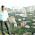2.I read in the Guardian recently (Chris Maslanka's puzzles) that no perfect square can leave a remainder of 3 or 5 on division by 7. No explanation or justification was given. Do you know of any?

1.Look at all possible remainders upon division by 7 (so 0 through 6) and see what the remainder is when you divide 0^2, 1^2, ..., 6^2 by 7. The possible remainders are 0, 1, 4, 2, 2, 4, and 1. So no perfect square gives a remainder of 3, 5, or 6 upon division by 7.

2.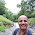679/7=97
Reminder is 0 but it is not perfect square number

3.It is not perfect square because, the last digit is 9(odd) and the penultimate digit is 7 which is also odd. The penultimate digit has to be an even number, to make the number perfect square.

4.What about 99976 which give previous digit to be 7odd ,on division it gives remainder to be 1

3.total number of prime factors is always even for a perfect square...total number of factors is odd...
ex:36=2^2 * 3^2
total no. of factors=(2+1)*(2+1)=6
total number of distinct prime factors=2(2,3)
correct me if i am wrong.thank you

1.just a small typo (2+1)*(2+1)=9 as u said it always has to be odd hence its true

4.5.According to these rules are 61 and 76 perfect squares

6.514 is a perfect square??...

It's digital root is 1

1.Nope, it's last 2 digits are not divisible by 4

7.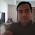8.81 = 9^2?
digital root is 9 so it fails on step 2
Is the digital root the iterative sum of the digits until there is only one digit or is it the remainder when the number is divided by 9?

1.Digital root is the sum of all digits to get one digit. 81 does not fail step two as its digital root is 9. Numbers with digital roots 2,3,7,8 are not perfect squares..

9.S siva:
514%7=3, so its not a perfect square.

10.what about the no like 10,1000. these numbers are not perfect squares. This method fails at step II.

1.You might not need to go to step 2 here. Step 1 rules out the possibility of perfect square as it ends with odd number of zeros.

11.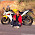is X64 perfect square??

12.It satisfies unit digit , digital root, unit digit 6 and tens place odd no, last two digit divisible by 4 and even no.
So where does ur method stand ?

13.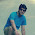100856 IS PERFECT SQUARE OR NOT ?

1. ENDS WITH 6
2. DIGITAL ROOT = 2
3. UNIT DIGIT =6 , AND TENS DIGIT IS ODD,
4. LAST TWO DIGIT 56, IS DIVISIBLE BY 4.

ANYONE CAN EXPLAIN ?

14.It's clearly stated that there are "riders" over and above these steps that these methods can only be used as a means of finding which number "cannot" be a perfect square ! Also, that even if one condition fails, the number fails to be a perfect square .. the conclusion sums it up perfectly -
" A number cannot be a perfect square if ....... "

15.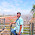15763530163289 is perfect square or not?

16.Is 250'and 5050 a perfect square

1.No because ends with odd number of zeroes

17.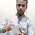1146600 it ends on 00, digital root is 9 but still it is not perfect square

1.after removing 00 you get 6 as unit digit but tens digit(6) is even
so it is not a perfect square.

18.Is there a method for binary numbers?

19.9981 is perfect square or not....can any one explain this correctly

20.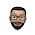Using this method we can only determine if a number is not a perfect square. That to only to an extent. You still need factorization to come to a final conclusion.

21.As per above explanation
step 1 satisfied
step 2 not satisfied. Digital root is 9.
But still it is a perfect square of 66
I dont think this concept will work always.

1.It satisfies. Digital root should be 1, 4, 7, or 9.

22.23.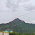A few numbers can't be checked by using this method.

24.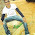According to your rules 99976 should be a perfect square but it is not a perfect square number

1.Some are saying this rule fails and all... common fellas..
This rule helps to find if a number is a imperfect square...
if a number let say 2356 satisfies all above rules, still we cant guarantee that the no is a perfect square. it only helps to channelise imperfect squares and not to tell that it is a perfect square

2.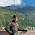This trick hasn't any sense... Not satisfying all conditions

25.184 is not a perfect square whil it's digital root is 4.
Can anybody tell me why???

26.If a perfect square is divisible by 4, but not 8, its remainder from 32 is always 4

27.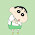i want reasons to show that 13,32,18,4487 are non perfect squares

28.Subtract 1 from given number and see that obtained number divisible by 3 or sum of digits of obtained number is 8, then the given number is perfect square.

29.1024 is a perfect square (of 32)
However digital root is 7. Explain?

30.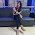What about the number 945729 ? Its digital root is 9 but its not a perfect square.How?

1.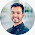we have two conditions.
1. if number ends with 2,3,7,8 its not an perfect square.
2. if digtal root is 1,7,4,9 the number may be a perfect square.
means if digital root is other than 1,7,4,9 we can say for sure that the number is not perfect square but the converse is not true

31.Square of even number not divisible by 4 leaves remainder 4 while square of an odd number always leaves remainder 1 when divided by 8?
what do you mean. plz explain with example

32.Square of even number not divisible by 4 leaves remainder 4 while square of an odd number always leaves remainder 1 when divided by 8?
what do you mean. plz explain with example

33.Please explain this :

If a number is divisible by 4, its square leaves a remainder 0 when divided by 8.

Square of even number not divisible by 4 leaves remainder 4 while square of an odd number always leaves remainder 1 when divided by 8.

34.Thank u sooooo much i am a student of 7th when I was in 6th there was nothing hard to search on internet but now suddenly I have a very hard course of maths subject and first time I am understood at first time and without any confusion now I'm the fan of this "Burning math"..... Again thanks I am vaishnavi. S. Singh

## Mental Math for Faster Calculation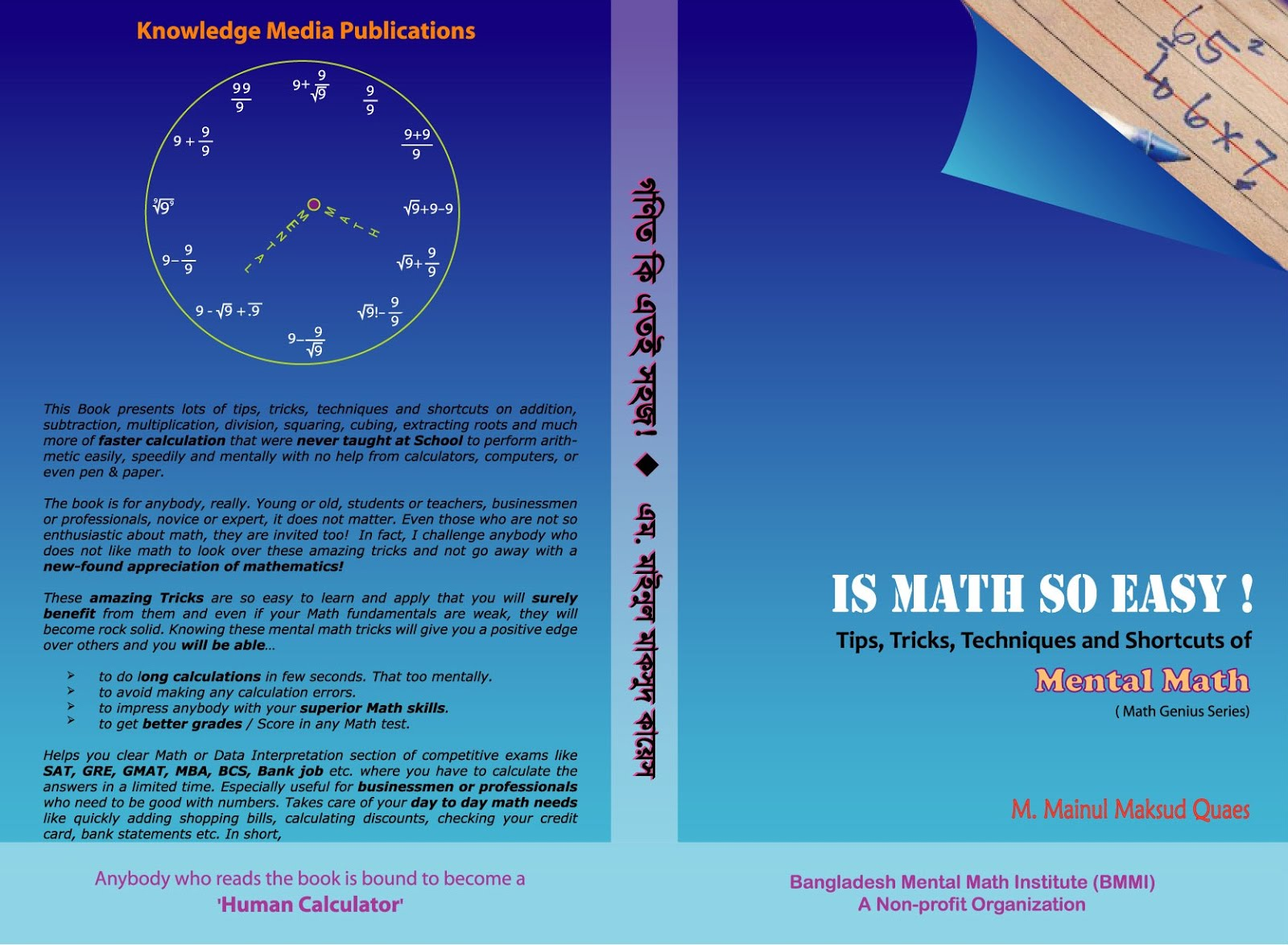© 2013-16 Burning Math